Download the eSaral app and start learning from Kota's top IITians and doctors.

# NCERT Solutions for Class 9 Maths chapter 7 Exercise 7.4 - Triangles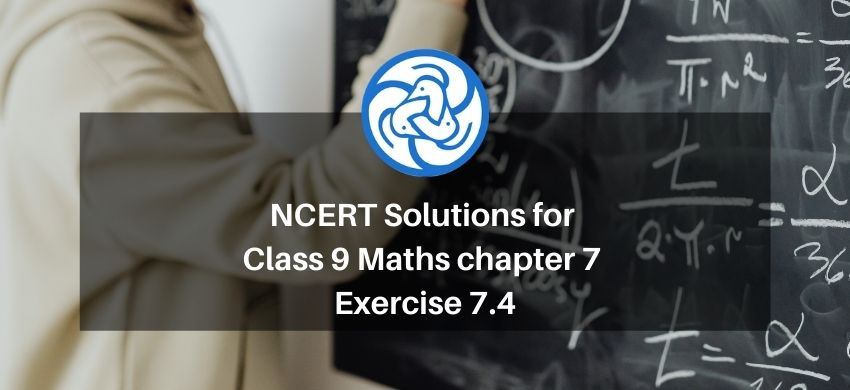Hey, are you a class 9 Student and Looking for Ways to Download NCERT Solutions for Class 9 Maths chapter 7 Exercise 7.4? If Yes then you are at the right place.

Here we have listed Class 9 maths chapter 7 exercise 7.4 solutions in PDF that is prepared by Kota’s top IITian’s Faculties by keeping Simplicity in mind.

If you want to score high in your class 9 Maths Exam then it is very important for you to have a good knowledge of all the important topics, so to learn and practice those topics you can use eSaral NCERT Solutions.

So, without wasting more time Let’s start.

### Download The PDF of NCERT Solutions for Class 9 Maths chapter 7 Exercise 7.4 "Triangles"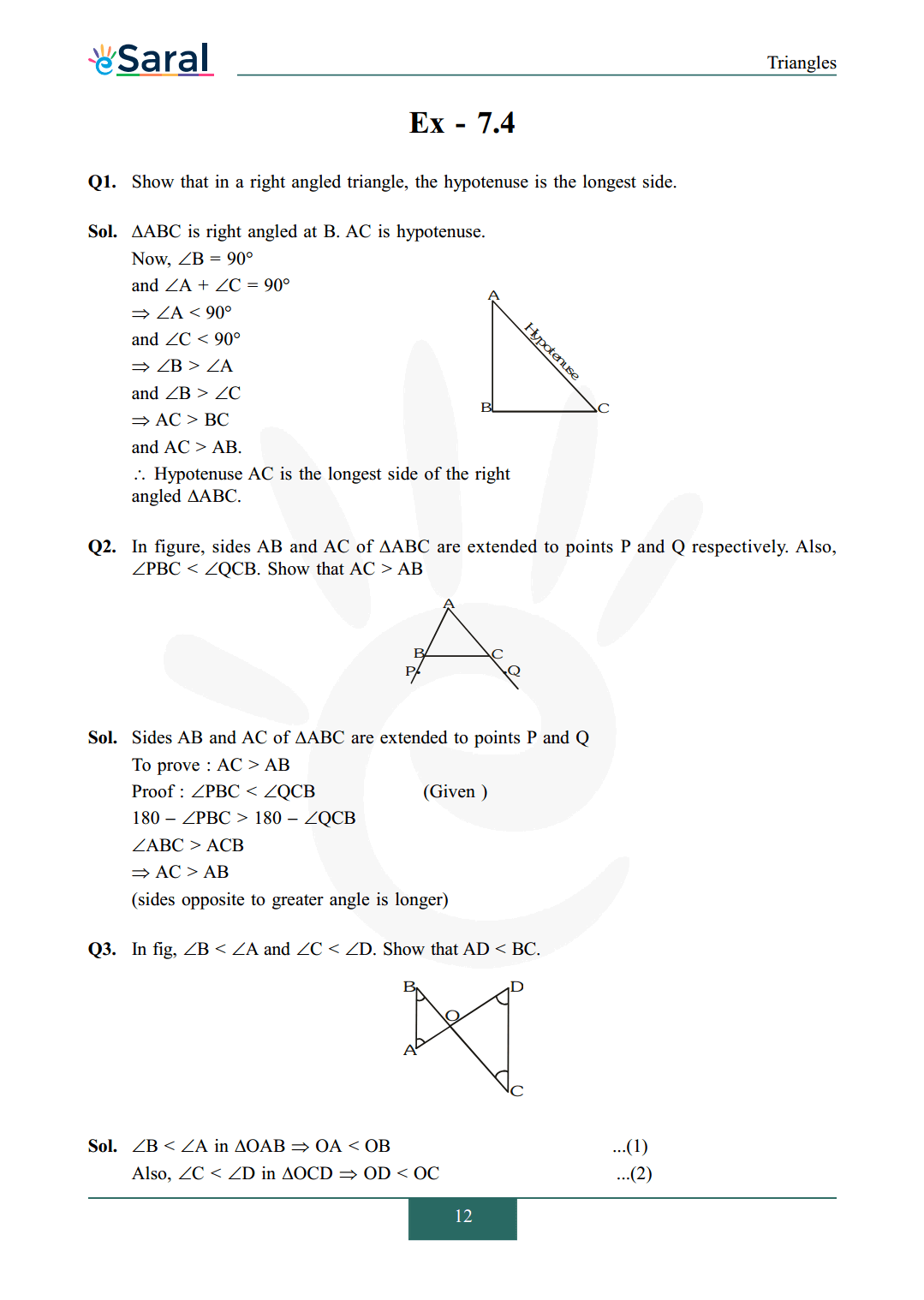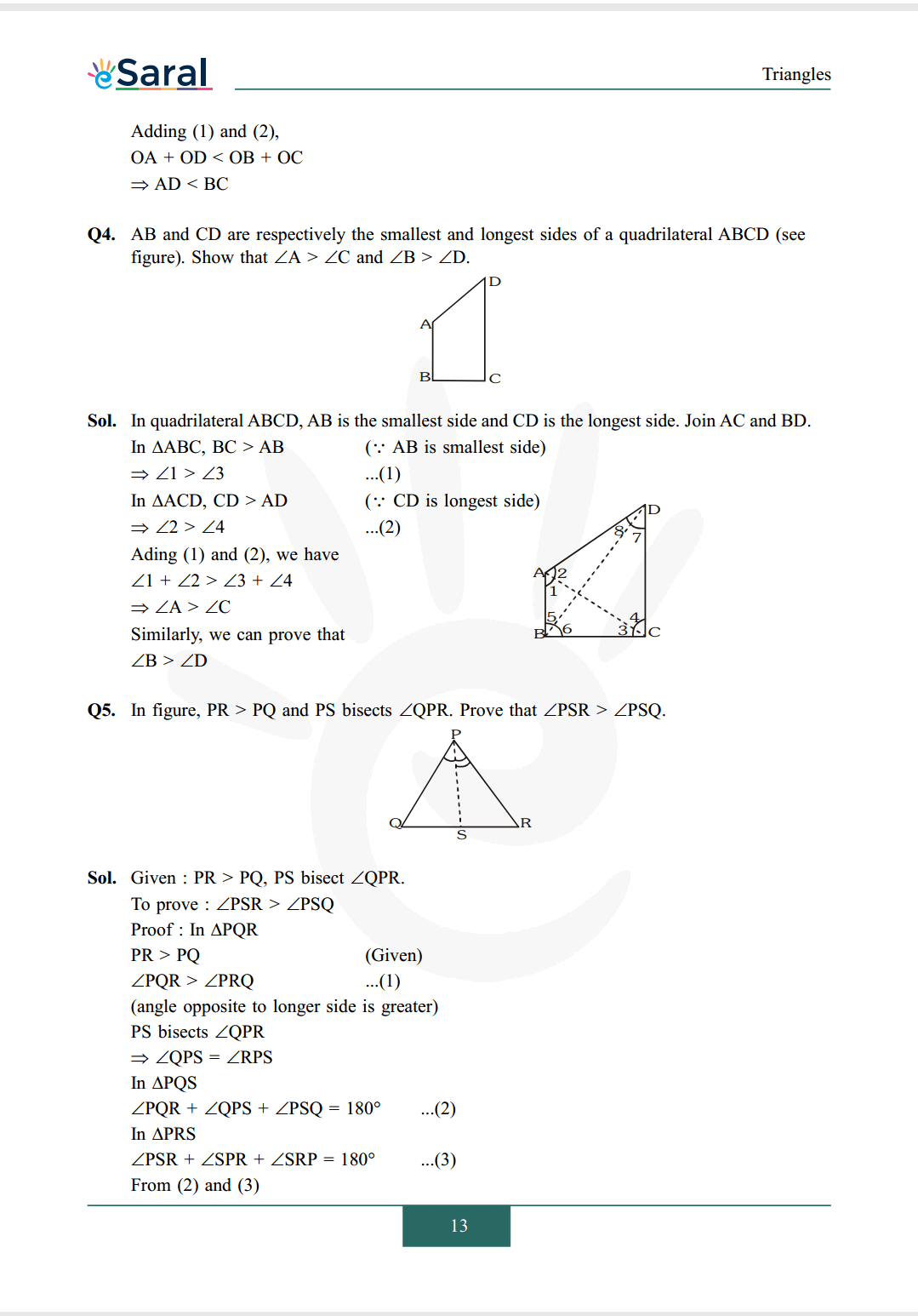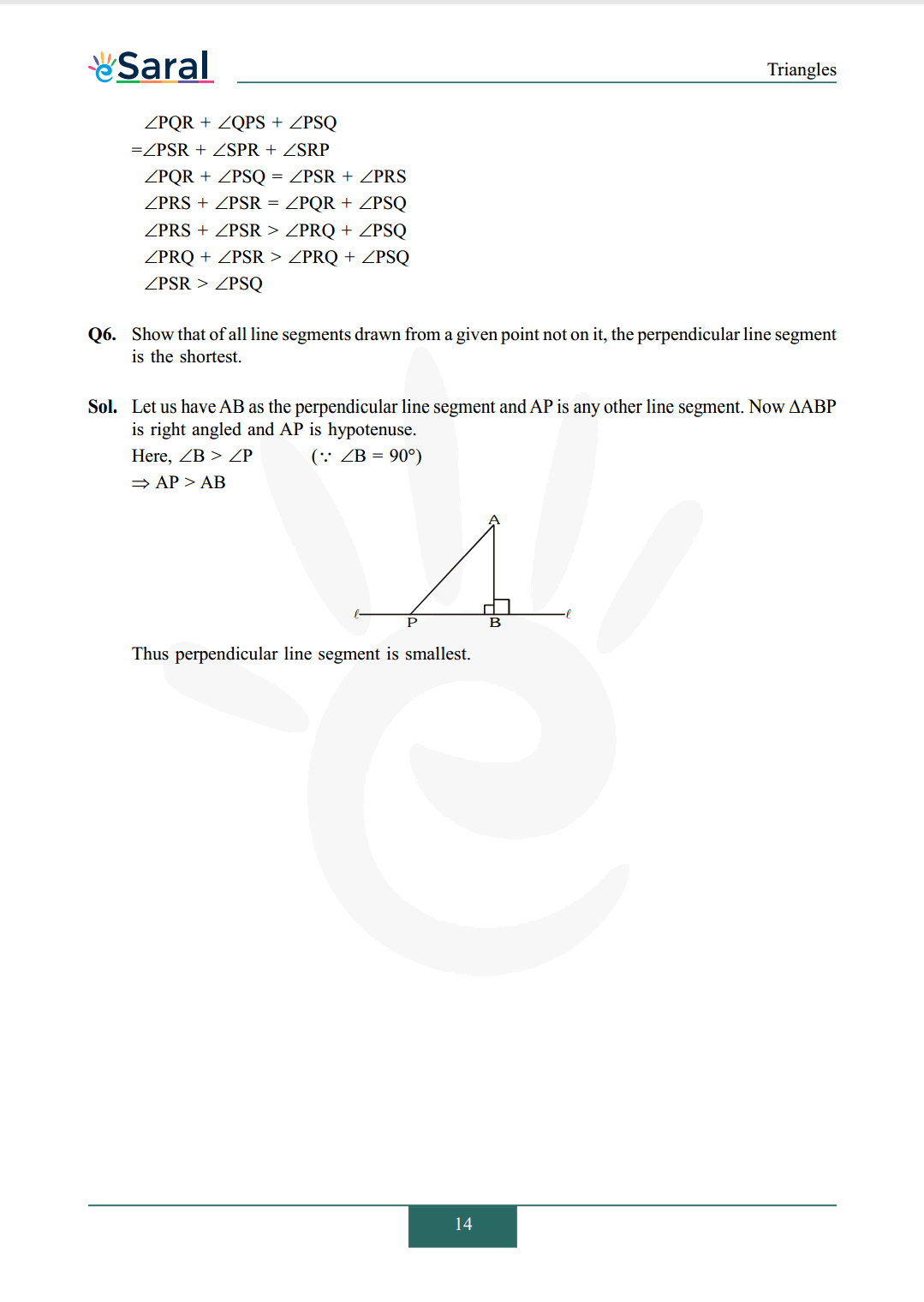#### All Questions of Chapter 7 Exercise 7.4

Once you complete the chapter 7 then you can revise Ex. 7.4 by solving following questions

Q1. Show that in a right angled triangle, the hypotenuse is the longest side.

Q2. In figure, sides $\mathrm{AB}$ and $\mathrm{AC}$ of $\Delta \mathrm{ABC}$ are extended to points $\mathrm{P}$ and $\mathrm{Q}$ respectively. Also, $\angle \mathrm{PBC}<\angle \mathrm{QCB}$. Show that $\mathrm{AC}>\mathrm{AB}$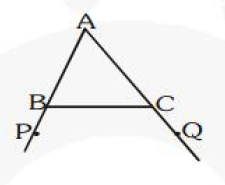Q3. In fig, $\angle \mathrm{B}<\angle \mathrm{A}$ and $\angle \mathrm{C}<\angle \mathrm{D}$. Show that $\mathrm{AD}<\mathrm{BC}$.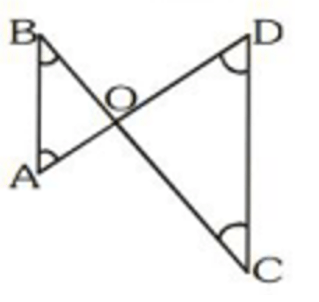Q4. $\mathrm{AB}$ and $\mathrm{CD}$ are respectively the smallest and longest sides of a quadrilateral $\mathrm{ABCD}$ (see figure). Show that $\angle \mathrm{A}>\angle \mathrm{C}$ and $\angle \mathrm{B}>\angle \mathrm{D}$.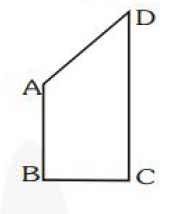Q5. In figure, $\mathrm{PR}>\mathrm{PQ}$ and $\mathrm{PS}$ bisects $\angle \mathrm{QPR}$. Prove that $\angle \mathrm{PSR}>\angle \mathrm{PSQ}$.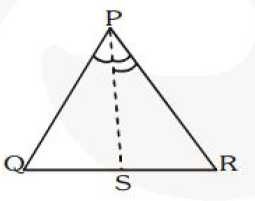Q6. Show that of all line segments drawn from a given point not on it, the perpendicular line segment is the shortest.

NCERT Class 9 Maths Book Chapterwise PDF

NCERT Class 9 Maths Exemplar Chapterwise PDF

Complete Solutions for Class 9 Maths chapter 7

Class 9 Maths Chapter 6 Exercise 6.1 Solutions PDF

Class 9 Maths Chapter 6 Exercise 6.2 Solutions PDF

Class 9 Maths Chapter 6 Exercise 6.3 Solutions PDF

If you have any Confusion related to NCERT Solutions for Class 9 Maths chapter 7 Exercise 7.4 then feel free to ask in the comments section down below.

To watch Free Learning Videos on Class 9 by Kota’s top Faculties Install the eSaral App